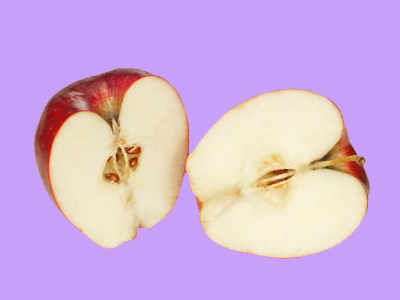If something is halved it is divided into two equally sized parts.

# Finding Halves

This Math quiz is called 'Finding Halves' and it has been written by teachers to help you if you are studying the subject at elementary school. Playing educational quizzes is a fun way to learn if you are in the 1st or 2nd grade - aged 6 to 8.

It costs only \$12.50 per month to play this quiz and over 3,500 others that help you with your school work. You can subscribe on the page at Join Us

Halving numbers or groups of objects is often young children’s first experience of fractions. They may relate this to cutting one object in two, dividing by two or sharing one group into two equal parts. Children should recognize that for halving to be accurate, both parts should be equal in size or amount.

Do you understand halves?

Question 1Cut some out
Share them into two equal groups
Put them into 4 piles
Put them in a bag
Each group should have exactly the same number of stars
Question 2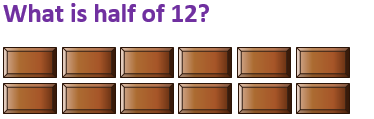6
12
14
24
12 ÷ 2 = 6, so half of 12 is 6
Question 3Cut it into 4 slices
Cut it into 2 equal parts
Share it with some friends
Eat some quickly
The two halves should be exactly the same size
Question 4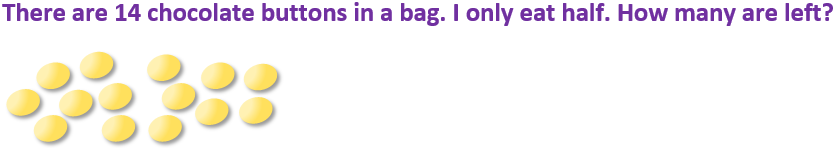7
6
5
10
Half of 14 is 7
Question 5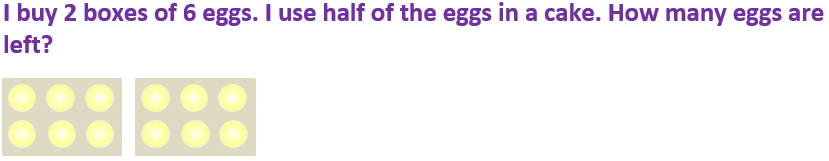2
16
6
4
Using half of the eggs would be the same as using one box only
Question 6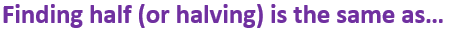Subtracting 2
Multiplying by 2
Dividing by 2
Halving means sharing into 2 equal groups or parts
Question 7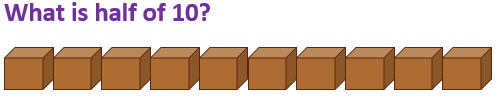8
5
20
35
10 shared into 2 equal groups gives 5 in each group, so half of 10 is 5
Question 8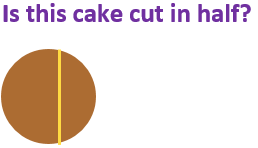Yes, there is a line down the middle
Yes, it has been shared between two people
Yes, there are two pieces
No, the two pieces aren’t the same size
Halves of a shape must be identical
Question 914
10
16
9
Half of 10 is 5, half of 8 is 4. 5 + 4 = 9
Question 1016
14
22
10
Sharing 20 into two equal groups would give 10 in each group
Author:  Angela Smith